View Course Path

# DC motor interfacing with 8051 using L293D and L298N

In this tutorial, we are going to interface a DC Motor with an 8051 microcontroller. By the end of this tutorial, we’ll learn:

• The DC motor – its working principle, pin descriptions, and how to change the direction of its rotation.
• What’s a motor driver and how important it is while interfacing with any microcontroller unit. You’ll learn two types of motor drivers: L298N and L293D.
• Two ways to control a motor:
• How to change the direction of rotation using switches.
• How to generate PWM logic using 8051 microcontrollers to control the speed of the motor.
• Develop the hardware/simulated circuit and write C code to implement the above.

Let’s start by making ourselves familiar with all the components that we’ll require for the entire process.

Contents

## Components Required

1. 8051 microcontroller – AT89C51/AT89S51/52 or similar variants.
2. Oscillator crystal – 11.0592Mhz
3. Capacitors – 22pF x 2, 10µF x 1
4. Resistors – 10kΩ x 3
5. Push-buttons or switches x 2
6. 12V DC battery or Adaptor
7. Motor driver – L293D H-Bridge
8. Motor driver – L298N H-Bridge
9. DC Motor x 1

## What is DC Motor?

A DC motor is a rotatory instrument that works on DC power to convert electrical energy into mechanical energy. DC motors can vary in size and power from small motors in toys and appliances to large mechanisms that power vehicles, pull elevators and hoists, and drive steel rolling mills, etc.The DC motor consists of the following components.

1. An outer stationary member called the stator.
2. The rotating member is called a rotor.
3. The clearance between the stator and rotor is called the air gap.
4. The rotor is mounted on the shaft and bearings where the bearings are fixed to the stator frame.
5. Both stator and rotor carry concentric cylindrical cores. Cores are laminated and made up of ferromagnetic materials to give optimum electric and magnetic characteristics.
6. When windings are excited, a common flux is set up. This flux crosses the air gap from one core to the other. N and S poles are produced on both stator and rotor. Such a magnetic system with alternate N and S poles is called a heteropolar system.
7. This stator and rotor move relative to each other for Electro-Mechanical Energy Conversion.

### How does a DC Motor work?

A DC motor consists of two terminals, and some of the modules available in the market do not indicate the polarity. However, reversing the connections of these terminals will change the direction of rotation of the motor.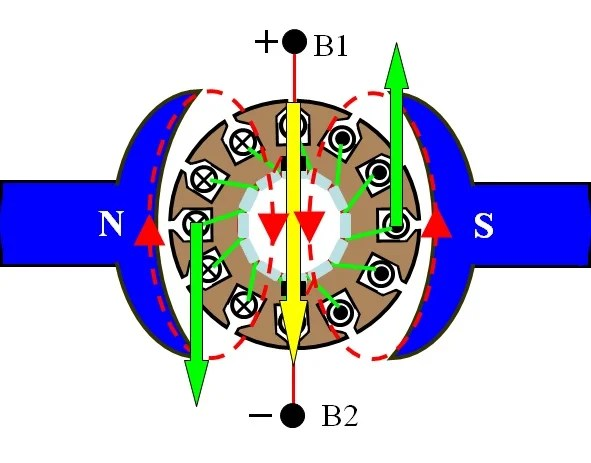When a DC supply is given to a DC motor in the way shown above:

• All conductors under N pole have a CROSS polarity.
• All conductors under S pole have DOT polarity.
• This produces stationary armature MMF in space from the positive terminal to the negative terminal.
• Therefore, a stationary or constant FORCE is applied to each conductor, and the direction of the force can be found out by using Fleming’s Left-Hand Rule.
• In this case, the rotor rotates in the anti-clockwise direction. The direction of rotation can be easily be reversed by interchanging the terminals.The two terminals of the DC motor can be connected in the following ways:

1. Terminal 1 connected to the positive terminal of DC supply & Terminal 2 is connected to a negative terminal or grounded: The shaft rotates in a clockwise direction.
2. Terminal 2 connected to the positive terminal of DC supply & Terminal 1 is connected to a negative terminal or grounded: The shaft rotates in an anti-clockwise direction.## What is an H-bridge?

The direction of flow of current determines the direction in which the DC motor rotates, i.e., clockwise or anti-clockwise. The H-bridge circuit provides a bi-directional flow of current through the armature of the DC motor, with the help of four switches, which are fashioned in such a way that looks like the letter ‘H.’ Hence, it is called an H-bridge circuit.

The H-bridge circuit eliminates the need to physically remove the battery and connect it in opposite polarity every time we want to change the direction of rotation. Check out the figure below:• When the switches S1 and S4 are ON and switches S2 and S3 are OFF, the current flows from A to AA, and the motor rotates in one direction, say clockwise.
• When the switches S2 and S3 are ON and switches S1 and S4 are OFF, the current flows from AA to A, and the motor rotates in the other direction, i.e., anti-clockwise.

We have the option to choose from two popular H-bridge ICs – L293D and L298N. The L298N IC is a dual H-bridge driver, whereas, the L293D IC is a quadruple high-current and half-H motor driver. Let’s check out what that means and how these ICs work.

Most DC motors have power requirements well out of the reach of a microcontroller. Moreover, the voltage spikes produced while reversing the direction of rotation can easily damage the microcontroller. Hence, it is not wise to connect a DC motor directly to the microcontroller. This is why we use a motor driver.

## H-bridge L293D Motor Driver

The L293D is designed to provide bidirectional drive currents of up to 600-mA at voltages from 4.5 V to 36 V. Every output is a complete totem-pole drive circuit, with a Darlington transistor sink and a pseudo-Darlington source. The enable pin enables the drivers in pairs, i.e., when EN1 is set HIGH, IN1 and IN2 are active and take the inputs from the microcontroller and give the outputs to the motor connected to OUT1 and OUT2. When the enable input is LOW, the drivers are disabled, and their outputs are OFF and are in the high-impedance state.

This motor driver is designed to drive inductive loads such as relays, solenoids, DC motors, and bipolar stepping motors, as well as other high-current/high-voltage loads in positive-supply applications.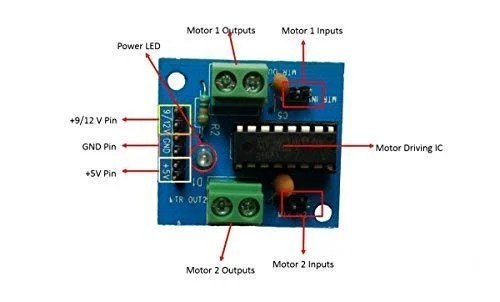Pin diagram of L293D ICWorking of L293D Motor driverRatings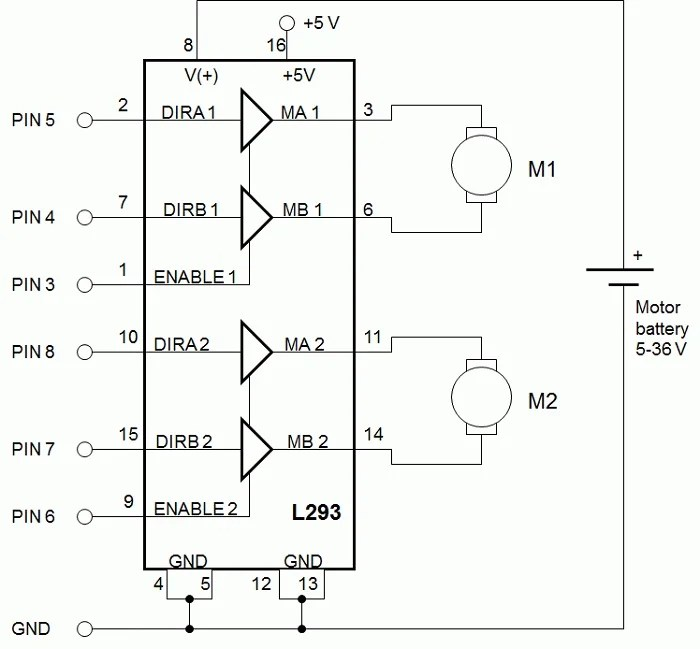Pin Pin Name Description 1 Enable 1,2 This pin enables the input pins: Input 1 (pin 2) and Input 2 (pin 7) 2 Input 1 Directly controls the Output 1 pin. 3 Output 1 Connected to one end of Motor 1 4 Ground Ground pins are connected to the ground of circuit (0V) 5 Ground Ground pins are connected to the ground of circuit (0V) 6 Output 2 Connected to another end of Motor 1 7 Input 2 Directly controls the Output 2 pin. 8 Vcc2 (Vs) Connected to Voltage pin for running motors (4.5V to 36V) 9 Enable 3,4 This pin enables the input pins: Input 3 (pin 10) and Input 4 (pin 15) 10 Input 3 Directly controls the Output 3 pin. 11 Output 3 Connected to one end of Motor 2 12 Ground Ground pins are connected to the ground of circuit (0V) 13 Ground Ground pins are connected to the ground of circuit (0V) 14 Output 4 Connected to another end of Motor 2 15 Input 4 Directly controls the Output 4 pin. 16 Vcc2 (Vss) Connected to +5V.

When the Enable 1 pin is HIGH, the inputs IN1 and IN2 take the inputs from the 8051 microcontroller. When two motors are connected to the L293D motor driver, then both the enable pins should be HIGH to make IN1, IN2, IN3, and IN4 get the inputs from the microcontroller.

• When both IN1 and IN2 are HIGH or LOW, the motor stays still as there is no potential difference.
• When IN1 is HIGH, and IN2 is LOW, then current flows from higher potential to lower potential. Therefore, the motor rotates in the clockwise direction.
• When IN1 is LOW, and IN2 is HIGH, then the current flow in the opposite direction. Therefore, the motor rotates in the anti-clockwise direction.
 EN1 = HIGH EN2 = HIGH IN1 IN2 OUT1 OUT2 Motor 1 IN3 IN4 OUT3 OUT4 Motor 2 HIGH LOW HIGH LOW Clockwise HIGH LOW HIGH LOW Clockwise LOW HIGH LOW HIGH Anti-clockwise HIGH LOW HIGH LOW Anti-clockwise HIGH HIGH HIGH HIGH Stays still HIGH HIGH HIGH HIGH Stays still LOW LOW LOW LOW Stays still LOW LOW LOW LOW Stays still
• Supply-Voltage Range: 5 V to 36 V.
• Output Current: 600 mA
• Logic voltage: 5V
• Internal ESD Protection.
• Thermal Shutdown.
• High-Noise-Immunity Inputs.
• Output Clamp Diodes for Inductive Transient Suppression.

## H-bridge L298N Motor Driver

The L298N Motor Driver Module is a high-power motor driver module which is used to drive DC and Stepper Motors. It consists of an in-built +5V voltage regulator. The L298N module can control up to four DC motors, or two DC motors with direction control and speed control because of its dual H-bridge circuit.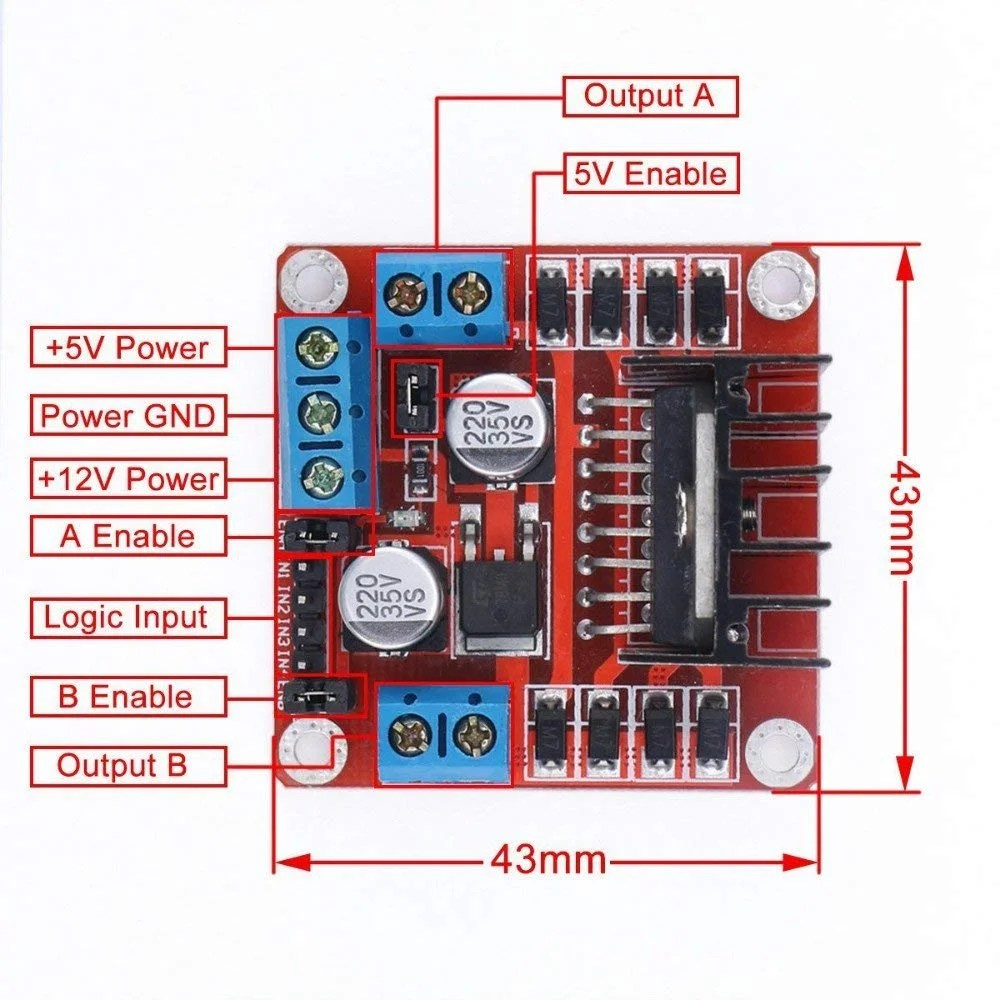Pin diagram of L298N ICWorking of L298N Motor driveRatings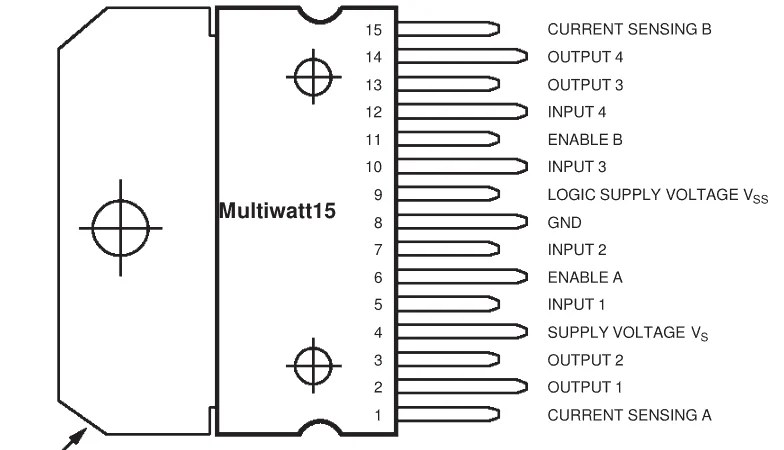Pin Pin Name Description 1 Sense A The sense resistor is connected between this pin and the ground to control the current to the motor. 15 Sense B 2 OUT1 Output pins of Motor A 3 OUT2 4 VS (5V) Supplies power for the switching logic circuitry inside L298N Motor driver IC. A non-inductive 100nF capacitor must be connected between this pin and ground. 5 INPUT 1/ IN1 Motor A input pins. It is used to control the direction of rotation of Motor A 7 INPUT 2/ IN2 6 ENA Enables the PWM signal for Motor A. 11 ENB Enables the PWM signal for Motor B. 8 GND Ground pin 9 VSS (12V) 12V input from DC power Source 10 INPUT 3/ IN3 Motor B input pins. It is used to control the direction of rotation of Motor B 12 INPUT 4/ IN4 13 OUT3 Output pins of Motor B 14 OUT4

If you’re using a motor of 12V rating, the supply voltage, Vs is connected to a battery of 12V, and the supply power for the switching circuitry is 5V, which is given to the Vss pin. The output pins have a potential difference of 12V, which is connected to the motor. When both the enable pins are HIGH, then based on the status of the input pins, the direction of the motor changes.

• IN1 = 1, IN2 = 0: Clockwise rotation
• IN1 = 0, IN2 = 1; Anti clockwise rotation
• IN1 = 0; IN2 = 0; No rotation
• IN1 = 1; IN2 = 1; No rotation
• Input voltage: up to 40V.
• Output current: up to 3A
• Power rating: up to 25W
• Drive voltage: 5-35V
• Logic voltage: 5V
• It consists of two built-in H-bridges, high voltage, large current, full-bridge driver, that can be used to drive DC motors, stepper motors, relay coils, and other inductive loads.
• We can further connect a high-capacity filter capacitor along with the free-wheeling diode connected in parallel with the switches that protect devices in the circuit from being damaged by the reverse current of an inductive load. Thus, enhancing its reliability.

## Using push-button switches to control the direction of rotation

Let’s take an example. The input pins IN1 and IN2 of the motor driver are connected to the pins P2.0 and P2.1, respectively, and we’re using the L293D motor driver to interface and control a DC motor with 8051 microcontrollers. We’ll send the logic shown below to Port 2 of the 8051 and provide a delay between switching from one direction to another.

 EN1 P2.1 / IN2 P2.0 / IN1 Motor Status High Low Low Stops High Low High Clockwise High High Low Anti-Clockwise High High High Stops

Here we’re going to use push-buttons to control the direction of the DC Motor. If you’re not familiar with the interfacing of a push-button with 8051 microcontrollers, feel free to check out this brief tutorial: Switch interfacing with 8051.

Here, the push-buttons are used in such a way that,

• When push-button 1 (switch_1) is ON (pressed), the input to IN1 (P2.0) is HIGH, and IN2 (P2.1) is LOW, which makes the motor rotate in the clockwise direction.
• When push-button 2 (switch_2) is ON (pressed), the input to IN1 (P2.0) is LOW, and IN2 (P2.1) is HIGH, which makes the motor rotate in the anti-clockwise direction.
• When both the push-buttons are ON, or both are OFF simultaneously, then the motor comes to standstill position/ remains in a standstill position.

In this way, we can interface the DC motor with 8051. Now, let’s look at the circuit diagram and thereon learn how to write the embedded C code to implement it.

### Circuit diagram

• Step 1: If you’re using Proteus or and other simulation software or even hardware, select the AT89C51 or AT89S51 microcontroller or any other compatible variant.
• Step 2: Connect a 12 MHz oscillator between pin 18 and 19.
• Step 3: Connect two capacitors of 22pF, with one terminal on either side of the oscillator and the other terminal to ground, as shown below.
• Step 4: Set Pin 31, i.e., EA pin to HIGH by connecting it to the +5V DC source.
• Step 5: Now, to make the RESET circuit, connect Pin 9 (RST) to +5V through a capacitor of 10µF and connect the same pin to +0V (GND) through a 10kΩ resistor or a potentiometer.
• Step 6: Here’s the interfacing part. Connect P2.0 and P2.1 of 8051 to IN1 and IN2 of the L293D motor driver. The connections from P2.6 and P2.7 to IN3 and IN4 are made only when you want to operate another motor through the output OUT3 and OUT4 of L293D.
• Step 7: Connect VSS to +5V supply and Vs to +12V supply and connect both the GND pins of L293D to ground.
• Step 8: Connect EN1 to +5V power supply and a DC motor between OUT1 and OUT2 pins of L293D IC.
• Step 9: To control the directions, connect two push-buttons to the pins P0.0 and P0.1, and code such that when P0.0 is pressed, the motor rotates in the clockwise direction and when P0.1 is pressed the motor rotates in the anti-clockwise direction, and when both are LOW or HIGH, the motor stops.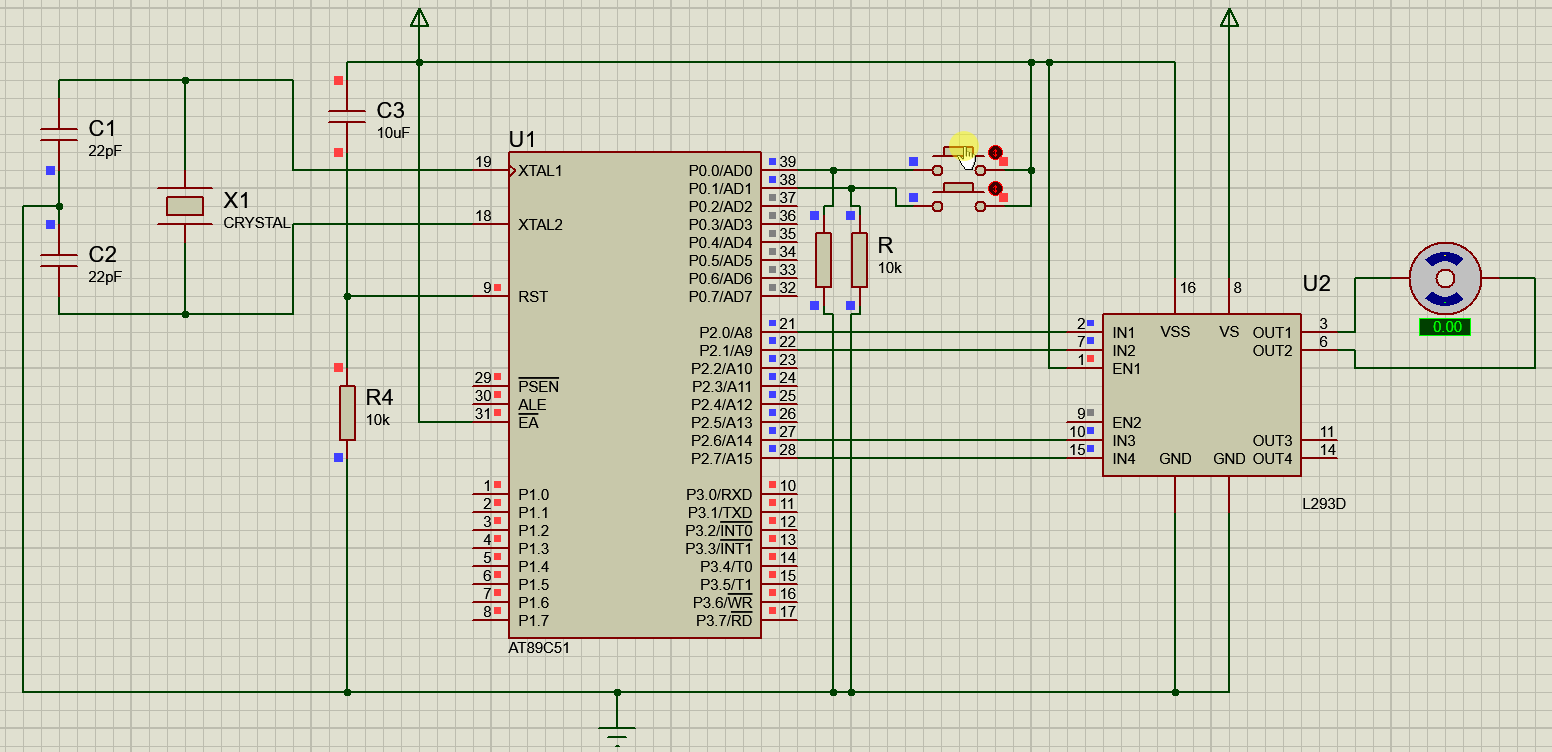### C Code

```#include<reg51.h>
sbit switch_1 = P0^0;
sbit switch_2 = P0^1;
sbit IN1 = P2^0;
sbit IN2 = P2^1;

void main()
{
P2 = 0x00; // to make P2 as output port
P0 = 0xFF; // to make P0 as input port

switch_1 = 0; // Initialize the push button 1
switch_2 = 0; // Initialize the push button 2

IN1 = 0; // Initialize with 'stop' logic
IN2 = 0; // Initialize with 'stop' logic

While (1) // Repeat this loop until stopped
{
if (switch_1 == 1) // If pushbutton 1 is pressed
IN1 = 1; // Rotate clockwise

else if (switch_2 == 1) // If Pushbutton 2 is pressed
IN2 = 1; // Rotate anti clockwise

else // If both are not pressed
P2=0x00; // Stop the motor
}
}```

## Using PWM technique to control the speed of the DC Motor

The Pulse Width Modulation (PWM) technique is a method of generating an analog signal using a digital source. A PWM signal consists of two main components that define its behavior: duty cycle and frequency.

• The duty cycle describes the amount of time the signal is in a high (on) state as a percentage of the total time it takes to complete one cycle.
• The frequency determines how fast the PWM completes a cycle (i.e., 1000 Hz would be 1000 cycles per second), and therefore, how fast it switches between high and low states.

Since we’re using a DC power supply, the output remains constant, in our case, 12V. But we can vary this voltage that is being fed to the motor by using pulse width modulation techniques. By increasing or decreasing the width of the ON pulse, we can control the speed of the DC motor. Since the speed of the DC motor is given by the formula

N = k(V – Ia x Ra) / ∅

N ∝ V

Where,

• k = proportionality constant
• N = speed of the motor in RPM
• V = Applied voltage
• Ia = Armature current
• Ra = Armature resistance
• ∅ = Flux per pole

Therefore, when V increases, speed increases too. But how can we vary the DC voltage? Isn’t it constant?

Simple. For a time period of 200us, if the DC voltage = 12V for the first 100us and 0V for the remaining 100us, then the average voltage in a cycle will be 6V. Let’s demonstrate this by using four push-buttons in such a way that:

• when PB1 is pressed, the duty cycle = 25%, therefore avg voltage to the motor  = 25% x 12V = 3V.
• when PB2 is pressed, the duty cycle = 50%, therefore avg voltage to the motor  = 50% x 12V = 6V.
• when PB3 is pressed, the duty cycle = 75%, therefore avg voltage to the motor  = 75% x 12V = 9V.
• when PB4 is pressed, the duty cycle = 100%, therefore avg voltage to the motor  = 100% x 12V =12V.#### How do we generate a pulse with desired duty cycles?

The answer is by using the built-in timers in 8051. A DC motor has a PWM frequency of 1-10kHz. For this example, the frequency is 5kHz, so the time period is 200us. We generate delays using timers of 8051 of the required duration.

 Time period = 200 us IN1 – P0.0 IN2 – GND Push-button Duty Cycle Ton Toff 1 0 PB1 – P3.0 25% 50 us 150 us 1 0 PB2 – P3.1 50% 100 us 100 us 1 0 PB3 – P3.2 75% 150 us 50 us 1 0 PB4 – P3.3 100% 200 us 0 us

Here are the steps to generate a time delay of 50 us using a timer of 8051 with an oscillator frequency of 11.0592Mhz. Feel free to brush up your concepts of timers and counters on how to generate delays here: Timers and Counters in 8051

• Step 1: Load TMOD register value i.e. TMOD = 0x01 to run Timer0 as a timer in Mode 1 (16-bit timer mode).
• Step 2: Load calculated TH0 value: TH0 = 0xFF.
• Step 3: Load calculated TL0 value: TL0 = 0xD2.
• Step 4: Start the timer by setting TR0 bit: TR0 = 1.
• Step 5: Wait till the TF0 flag overflows.
• Step 6: Stop the timer by clearing TR0 bit: TR0 = 0.
• Step 7: Clear timer flag TF0 bit: TF0 = 0.

The formula used to find out the values of TH0 and TL0 is: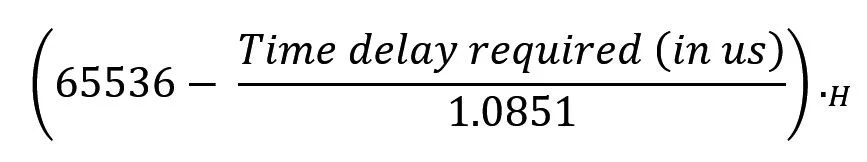For a delay of 50us,

65536 – (50/1.0851) = 65490D = FFD2H

Therefore, TH0 = 0xFF and TL0 = 0xD2

In the same way, you can find out for other duty cycles as well. So, to get a duty cycle of 25%, pin 0.0 (connected to IN1) is set to ‘1’ for 50us and ‘0’ for 150us in one cycle of time period T = 200us.

Note that here we’re using L298N driver as it has higher ratings and can handle high rated DC motors as well. But you can also use the L293D motor driver.

Now that you’ve understood the logic behind the speed control of DC motor using PWM signals generated by 8051 let’s look at the circuit diagram and how to code the functionality using embedded C.

### Circuit Diagram

The first few steps are the same as the ones we saw while interfacing the L293D. Let’s skip those steps and move onto interfacing the DC motor using the L298N.

• Step 1: Connect four push-buttons to pins P3.0, P3.1, P3.2, and P3.3.
• Step 2: We connect pin P0.0 to IN1 of L298N motor driver, and IN2 is to be connected to GND, to make sure that the motor rotates in a single direction.
• Step 3: ENA and VCC pins are connected to the +5V supply and GND pin to the ground. Connect Vs to a 12V battery (depends on the rating of the motor, if the motor is rated 25V, then connect a 25V battery).
• Step 4: The motor is connected between OUT1 and OUT2 pins of the L298N motor driver.
• Step 5: A resistor can be connected between Sense A and Sense B pins to control or limit the output current to the motor, which is up to 80mA.### C Code

```#include<reg51.h>
sbit IN1 = P0^0;
sbit PB1_Duty_25 = P3^0;
sbit PB2_Duty_50 = P3^1;
sbit PB3_Duty_75 = P3^2;
sbit PB4_Duty_100 = P3^3;

void PWM(void);
void PWM()
{
TR0 = 1; // Start the timer
while (TF0 == 0); // Wait till the timer flag overflows
TR0 = 0; // Stop the timer
TF0 = 0; // Reset the flag
}

void main()
{
// Declarations
P0 = 0x00; // Configure Port 0 as an output port
P3 = 0xFF; // Configure Port 3 as an input port

TMOD = 0x01; //Timer-0, As 16-bit Timer (Mode 1).

while(1) // Repeat this loop until stopped
{
if (PB1_Duty_25 == 0) // If PB1 is pressed duty_cycle = 25% should be set
{
TH0 = 0xFF;
TL0 = 0xD2;
IN1 = 1; // Pulse = HIGH
PWM(); // For 50 us

TH0 = 0xFF;
TL0 = 0x76;
IN1 = 0; // Pulse = LOW
PWM(); // For 150 us
}

if (PB2_Duty_50 == 0) // If PB2 is pressed duty_cycle = 50% should be set
{
TH0 = 0xFF;
TL0 = 0xA4;
IN1 = 1; // Pulse = HIGH
PWM(); // For 100 us

TH0 = 0xFF;
TL0 = 0xA4;
IN1 = 0; // Pulse = LOW
PWM(); // For 100 us
}

if (PB3_Duty_75 == 0) // If PB 3 is pressed duty_cycle = 75% should be set
{
TH0 = 0xFF;
TL0 = 0x76;
IN1 = 1; // Pulse = HIGH
PWM(); // For 150 us

TH0 = 0xFF;
TL0 = 0xD2;
IN1 = 0; // Pulse = LOW
PWM(); // For 50 us
}

if (PB4_Duty_100 == 0) // If PB4 is pressed duty_cycle = 100% should be set
{
TH0 = 0xFF;
TL0 = 0x48;
IN1 = 1; // Pulse = HIGH
PWM(); // For 200 us

TH0 = 0xFF;
TL0 = 0xFF;
IN1 = 0; // Pulse = LOW
PWM(); // For 0 us
}
}
}
```

This site uses Akismet to reduce spam. Learn how your comment data is processed.